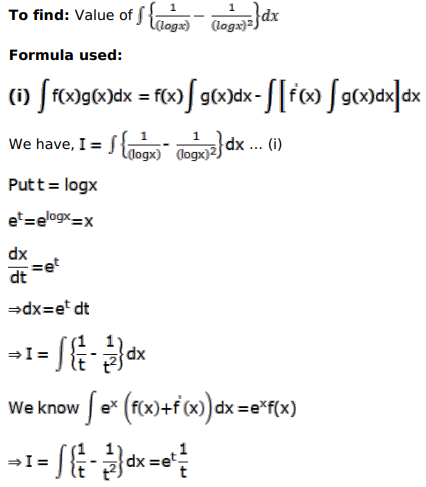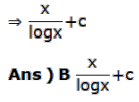# Mark against the correct answer in each of the following:

Question:

Mark $(\sqrt{ })$ against the correct answer in each of the following:

$\int\left\{\frac{1}{(\log x)}-\frac{1}{(\log x)^{2}}\right\} d x=?$

A. $\times \log x+C$

$B .$ $\frac{x}{\log x}+C$

D. none of these

Solution: